NewsEtc.

# Diffuse reflectance from a semiinfinite medium

May 17, 1999, Steven L. Jacques

When photons enter a tissue, they scatter and escape out the surface where they are observable. The escaping flux of light is called "diffuse reflectance", Rd. The value of Rd depends on the absorption coefficient of the tissue, µa, and the effective pathlength L that photons travel in the tissue.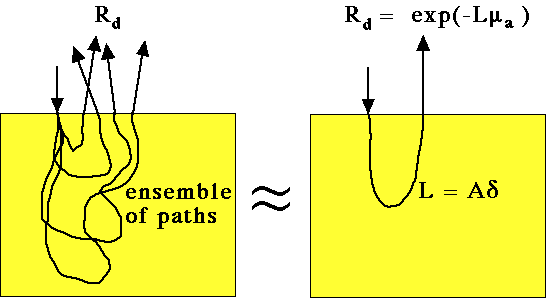Rd is uniquely related to the ratio of scattering to absorption. The more scattering events that occur before the photon is absorbed, the more likely is the photon to be returned to the surface for escape as observable reflectance. If both the absorption and the reduced scattering coefficients are doubled or halved, the Rd does not change because the ratio of scattering to absorption remains constant.

The diffuse reflectance from the surface of a semi-infinite homogeneous medium with absorption coefficient µa [cm-1] and reduced scattering coefficient coefficient µs' [cm-1] is approximated by the expression: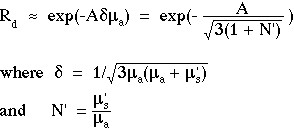is the 1/e optical penetration depth. N' is defined as the ratio of reduced scattering to absorption. The factor Aequals the apparent pathlength L for photon attenuation due to the absorption coefficient µa. In other words, the ensemble of photon pathlengths for escaping light observed as Rd can be approximated by a single pathlength L = A.

The following figure shows the behavior of Rd for an aqueous solution, in other words for a refractive index mismatch of 1.33:1 at the air/medium surface: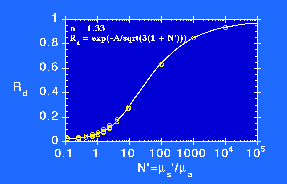for air/water surface with refractive index mismatch 1.33:1.

which is based on calculations using the Adding-Doubling Method. The data are for various choices of choices of absorption and reduced scattering and for various choices of anisotropy, g = 0, 0.5, 0.8, 0.9, 0.95. Above Rd = 0.40, g has no influence on Rd. Below Rd = 0.40, the Rd depends on the anisotropy.

The value of A is not constant, and depends on N' and the refractive index mismatch at the air/medium surface. The value of A is roughly about 7-8 for most soft tissues, and choosing a constant value of A allows Eq. 1 to roughly mimic the behavior of Rd. For an accurate calculation of Rd, however, the appropriate value of A should be specified as a function of N':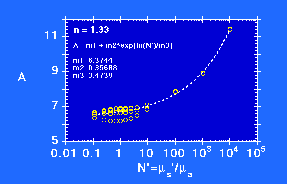for air/water surface with refractive index mismatch 1.33:1.

In summary, Rd is a function of µs'/µa = N'.

## Deduce N' from a measurement of Rd

A measurement of Rd is perhaps the simplest measurement one can make on a semi-infinite scattering medium. The following figure illustrates how measurements on the unknown medium (M) and on a standard reflectance (Mstd) whose reflectance is Rstd yield Rd: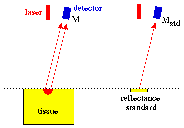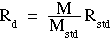Once Rd is specified, one can calculate N':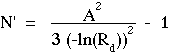Since A is a function of N', one must use an iterative loop, or a fitting routine, to specify both A and N' from a given Rd. N' and A are not independent, so we are still specifying only one unknown, N', from one independent variable, Rd. A simple MATLAB program illustrates such an iterative loop: N' = findNp(Rd).

May 99 issue | News Etc. index | Home